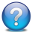## Pages

### The Binomial Distributon

The Binomial Distribution

• It is a discrete probability distribution.
• The distribution of a random variable X is discrete, if it can assume only a finite or countably infinite number of values.
• Considering u the set of all possible values of X: $$\sum_u Pr \left(X = u\right) = 1$$
• The binomial distribution is the discrete probability distribution of the number of successes in a sequence of n independent yes/no experiments, each of which yields success with probability p.
• Each success/failure experiment is called a Bernoulli trial.
• The binomial distribution is the basis of the binomial test of statistical significance
• It is frequently used to model the number of successes in a sample of size n drawn with replacement from a population of size N. The replacement after each draws makes the draws independents.

If the probability of a successful trial is p, then the probability of having exactly k successes in n identical independent trials is given by the probability mass function below:
\begin{aligned} f\left(k; n,p \right) = Pr \left(X = k\right) = \binom{n}{k} p^k {\left( 1 - p \right)}^{n-k} \\ \text{for k = 0, 1, 2, ..., n, where} \\ \binom{n}{k} = \frac{n!}{k!(n-k)!} \end{aligned}

The formula can be understood as follows: we want k successes (with probability $p^k$) and n-1 failures (probability ${\left( 1 - n \right)}^{n-k}$). However, the k successes can occur anywhere among the n trials, and there are $\binom{n}{k}$ different ways of distributing k success in a sequence of n trials.Consider the following problem: One six-sided dice is rolled 15 times. What is the probability of rolling 5 or less 2's?
In each roll, the probability of rolling a particular number, say 2, is 1/6.
The probability of rolling 5 or less 2's is the sum of probabilities of rolling 0,1,2,3,4 and 5 2's.
\begin{aligned} Pr \left(X \leq 5\right) = \sum_{k=0}^5 Pr \left(X = k\right) \end{aligned}
Using R density or probability function dbinom() to obtain the probability:
• dbinom() returns the probability of an outcome of a binomial distribution
• The probability of rolling exactly 5 2's is
> dbinom(5, size=15, prob=0.167)
 0.06274624

• The probability of rolling 0,1,2,3,4 or 5 2's:
> dbinom(0, size=15, prob=0.167) +
+ dbinom(1, size=15, prob=0.167) +
+ dbinom(2, size=15, prob=0.167) +
+ dbinom(3, size=15, prob=0.167) +
+ dbinom(4, size=15, prob=0.167) +
+ dbinom(5, size=15, prob=0.167)
 0.9723556

• Alternatively, we can use the cumulative probability function for binomial distribution pbinom().
• $Pr\left(X \leq 5 \right)$
> pbinom(5,size=15, prob=0.167)
 0.9723556


• As seen above, the pbinom() function is useful to summing consecutive binomial probabilities.
• Other questions that can be answered include:
• What is the probability of rolling 5 or more 2's? $Pr\left(X \geq 5 \right)$
• $Pr\left(X \geq 5 \right) = 1 - Pr\left(X \leq 4 \right) = 1 - \text{pbinom(4, size=15, prob=0.167) = 0.09039}$
• > 1 - pbinom(4, 15, 0.167)
 0.09039063

• What is the probability of rolling more than 4 and less than 8 2's? $Pr\left(4 \leq X \leq 8 \right)$
• $Pr\left(4 \leq X \leq 8 \right) = Pr\left(X \leq 8 \right) - Pr\left(X \leq 5 \right) = \text{pbinom(8, size=15, prob=0.167) - pbinom(5, 15, 0.167) = 0.02720835}$
• > pbinom(8, 15, 0.16667) - pbinom(5,15, 0.16667)
 0.02720835


### Probability Distributions (I)

Probability distributions

• A probability distribution describes how the values of a random variable are distributed.
• It assigns a probability to each possible outcome of a process or experiment that is assumed random. The random variable can be continuous or discrete.
• Probability distributions can be very useful because, since the characteristics of each distribution are well understood, they can be used to, using a sample of observations, make statistical inferences on the entire population.
• A probability distribution can be specified in a number of ways:
• Through a probability density function (probability mass function)
• Through a cumulative distribution function (survival function)
• Through a hazard function
• Through a characteristic function
• Some common distributions include:
• Binomial distribution: dbinom()
• The collection of possible outcomes of a coin toss [H|T] follow a
• Cauchy distribution: dcauchy()
• Chi-squared distribution: dchisq()
• Exponential distribution: dexp()
• F distribution: df()
• Gamma distribution: dgamma()
• Hypergeometric distribution: dhyper()
• Log-normal distribution: dlnorm()
• Geometric distribution: dgeom()
• Multinomial distribution: dmultinom()
• Negative binomial distribution: dnbinom()
• Normal distribution: dnorm()
• Poisson distribution: dpois()
• Student's t distribution: dhyper()
• Uniform distribution: dunif()
• Weibull distribution: dweibull()

### If you get: "xterm-256color: Unknown terminal type"

When connecting to a Solaris host, using ssh, from MacOSX or a Linux machine, you may get the message when you try to use vi:

For example, you may try:
$vi instructions.txt xterm-256color: Unknown terminal type I don't know what kind of terminal you are on - all I have is 'xterm-256color'. [Using open mode] "instructions.txt" 25 lines, 2465 characters Instructions to run the Gateway Management utilitySolution? You need to define the TERM environment variable. $ export TERM=xterm
\$ vi instructions.txt